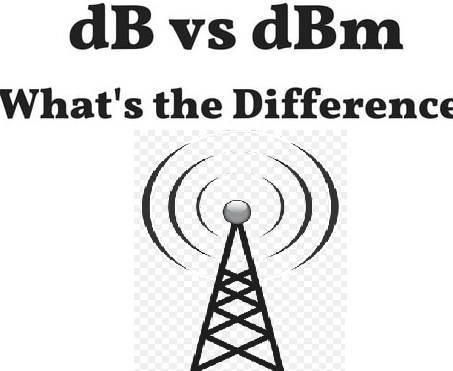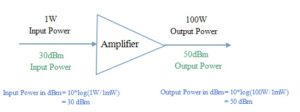# dB , dBm Terminology

I keep seeing people using the terms “dB” and “dBm”, interchangeably, when they actually mean very different things. So, here’s a little background on the correct usage of the terms.

All the zeros before the decimal point for high powers or after the decimal point for low powers make calculations difficult. For convenience, the dB and dBm system of units is used. (All microwave equipment performance is specified in dB and dBm, and all microwave measurement equipment have their scales calibrated in dB and dBm.)

Decibel (dB), is a specific function that operates on a unit-less parameter.

dB=10*log10 (x)

Where x is unitless!

For example, amplifier gain is a unit-less value i.e. amplifier gain is the ratio of the output power to the input power:

Pout/Pin = Gain

Gain in dB = 10*log(Pout/Pin)

Example:So, dB is a RELATIVE measure of two different POWER levels. There’s also dB relative to VOLTAGE levels, but I won’t go into those, as we’re mostly concerned with POWER levels in our discussions here. 3dB is twice (or half) as much, 6dB is four times, 10dB is ten times, and so on.

Thus, I can say that an amplifier has 20 dB of gain, or I have 6dB total feed-line cable loss. I CANNOT say, My amplifier gives output power of 30 dB, as you must state what you’re referencing it to, which is where the subscript comes in. The dB by itself is not an absolute number, but a ratio.

dBm is a (logarithmic) unit to measure powers. Since dB is a ratio of powers, dBm is defined by forming the ratio of the power you want to express relative to a reference power, which is 1 mW for dBm. Formally speaking,

Power in dBm = 10*log10 (Power / 1mW)

For amplifiers, a common reference unit of absolute power is the dBm, with 0dBm being equal to 1 milliwatt.

Example:Thus, an amplifier with an output power of 50dBm puts out 100 Watt. How much gain it has is a different matter entirely.